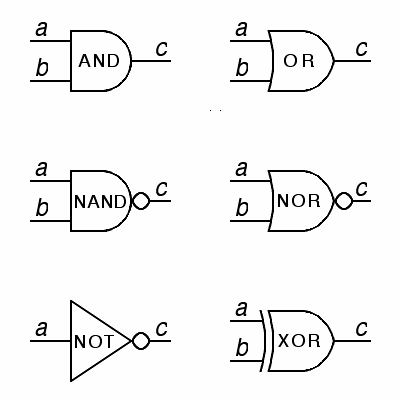Logic Gates & Circuits
3 years ago
timocracy
Save
Edit
Host a game
Live GameLive
Homework
Solo Practice
Practice12 QuestionsShow answers
• Question 1
30 secondsQ. Which logic gate is this?
AND
NOR
NOT
OR
• Question 2
30 secondsQ. Which logic gate is this?
AND
OR
XOR
NOT
• Question 3
30 secondsQ. Which logic gate is this?
XOR
NOR
NAND
NOT
• Question 4
30 secondsQ. Which logic gate is this?
OR
NOR
XOR
NXOR
• Question 5
30 secondsQ. Which logic gate is this?
NAND
NOR
XOR
NXOR
• Question 6
30 secondsQ. Which logic gate is this?
NOR
XOR
NXOR
NAND
• Question 7
30 secondsQ. Which logic gate is this?
NXOR
NAND
XOR
NOR
• Question 8
60 secondsQ. Which truth table is this?
NOT
AND
OR
NAND
• Question 9
60 secondsQ. Which truth table is this?
AND
OR
NOT
NOR
• Question 10
60 secondsQ. Which truth table is this?
OR
NOR
XOR
AND
• Question 11
60 secondsQ. Which truth table is this?
XOR
NAND
NOR
NOT
• Question 12
60 secondsQ. Which truth table is this?